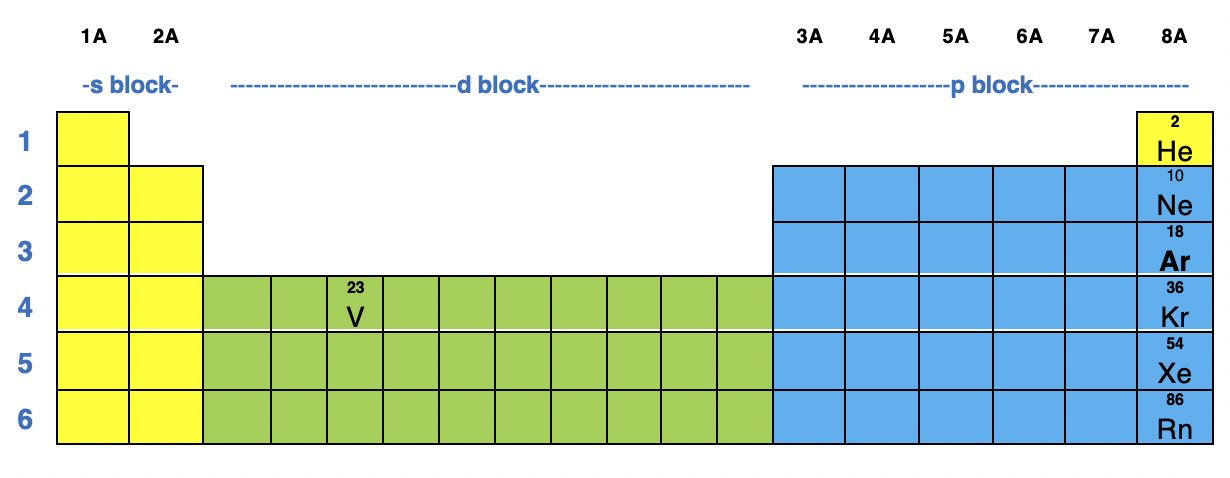# Problem: How many unpaired electrons would you expect on Vanadium in V2O3

###### FREE Expert Solution

Step 1: Recall that for a neutral element, Atomic number = # of protons = # of electrons

The atomic number of V is 23 which means V has 23 electrons.

The location of V in the periodic table is shown below:Based on the figure, V belongs in the d-block in Period 4 so its electron configuration will contain the 4s and 3d subshells

Recall that:

s–subshell can hold a maximum of 2 electrons

p–subshell can hold a maximum of 6 electrons

d–subshell can hold a maximum of 10 electrons

f–subshell can hold a maximum of 14 electrons

Starting from 1s, the electron configuration for V is:

V: 1s22s22p63s23p64s23d3

Step 2: In V2O3, the charge of V is +3.

The +3 charge means 3 e were lost. Therefore, we need to subtract 3 e starting from the highest energy level (4s).

The electron configuration for V3+ is:

V3+: 1s22s22p63s23p63d2

93% (282 ratings)###### Problem Details

How many unpaired electrons would you expect on Vanadium in V2O3